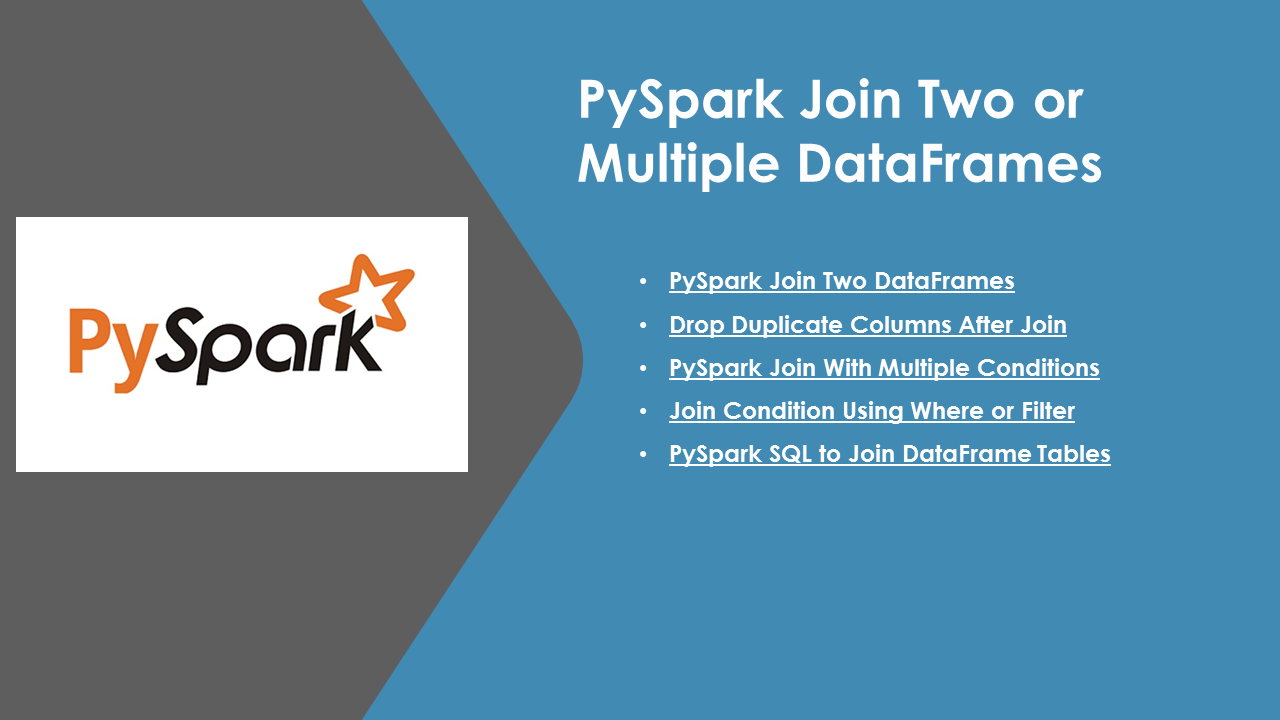# PySpark Join Two or Multiple DataFrames

PySpark DataFrame has a `join()` operation which is used to combine columns from two or multiple DataFrames (by chaining join()), in this article, you will learn how to do a PySpark Join on Two or Multiple DataFrames by applying conditions on the same or different columns. also, you will learn how to eliminate the duplicate columns on the result DataFrame and joining on multiple columns.

Note: Join is a wider transformation that does a lot of shuffling, so you need to have an eye on this if you have performance issues on PySpark jobs.

In order to explain join with multiple DataFrames, I will use Inner join, this is the default join and it’s mostly used. Inner Join joins two DataFrames on key columns, and where keys don’t match the rows get dropped from both datasets.

Before we jump into PySpark Join examples, first, let’s create an `emp` , `dept`, `address` DataFrame tables.

Emp Table

``````
empData = [(1,"Smith",10), (2,"Rose",20),
(3,"Williams",10), (4,"Jones",30)
]
empColumns = ["emp_id","name","emp_dept_id"]
empDF = spark.createDataFrame(empData,empColumns)
empDF.show()
``````

Dept Table

``````
deptData = [("Finance",10), ("Marketing",20),
("Sales",30),("IT",40)
]
deptColumns = ["dept_name","dept_id"]
deptDF=spark.createDataFrame(deptData,deptColumns)
deptDF.show()
``````

``````
(2,"3453 Orange St","SFO","NY"),
(3,"34 Warner St","Jersey","NJ"),
(4,"221 Cavalier St","Newark","DE"),
(5,"789 Walnut St","Sandiago","CA")
]
``````

## PySpark Join Two DataFrames

``````
join(right, joinExprs, joinType)
join(right)
``````

The first join syntax takes, `right` dataset, `joinExprs` and `joinType` as arguments and we use `joinExprs` to provide a join condition.

The second join syntax takes just the right dataset and joinExprs and it considers default join as `inner join`.

``````
//Using Join expression

#Result
+------+--------+-----------+------+---------------+------+-----+
+------+--------+-----------+------+---------------+------+-----+
|     1|   Smith|         10|     1|   1523 Main St|   SFO|   CA|
|     3|Williams|         10|     3|   34 Warner St|Jersey|   NJ|
|     2|    Rose|         20|     2| 3453 Orange St|   SFO|   NY|
|     4|   Jones|         30|     4|221 Cavalier St|Newark|   DE|
+------+--------+-----------+------+---------------+------+-----+
``````

This joins `empDF` and `addDF` and returns a new DataFrame.

## Drop Duplicate Columns After Join

If you notice above Join DataFrame `emp_id` is duplicated on the result, In order to remove this duplicate column, specify the join column as an array type or string. The below example uses array type.

Note: In order to use join columns as an array, you need to have the same join columns on both DataFrames.

``````
#Removes duplicate columns

#Result
+------+--------+-----------+---------------+------+-----+
+------+--------+-----------+---------------+------+-----+
|     1|   Smith|         10|   1523 Main St|   SFO|   CA|
|     3|Williams|         10|   34 Warner St|Jersey|   NJ|
|     2|    Rose|         20| 3453 Orange St|   SFO|   NY|
|     4|   Jones|         30|221 Cavalier St|Newark|   DE|
+------+--------+-----------+---------------+------+-----+
``````

PySpark `join()` doesn’t support join on multiple DataFrames however, you can chain the `join()` to achieve this.

``````
#Join Multiple DataFrames by chaining
.join(deptDF,empDF["emp_dept_id"] == deptDF["dept_id"]) \
.show()

#Result
+------+--------+-----------+---------------+------+-----+---------+-------+
+------+--------+-----------+---------------+------+-----+---------+-------+
|     1|   Smith|         10|   1523 Main St|   SFO|   CA|  Finance|     10|
|     3|Williams|         10|   34 Warner St|Jersey|   NJ|  Finance|     10|
|     4|   Jones|         30|221 Cavalier St|Newark|   DE|    Sales|     30|
|     2|    Rose|         20| 3453 Orange St|   SFO|   NY|Marketing|     20|
+------+--------+-----------+---------------+------+-----+---------+-------+
``````

## Join Condition Using Where or Filter

Let’s see a Join example using DataFrame `where()`, `filter()` operators, these results in the same output, here I use the Join condition outside join() method.

``````
#Using Where for Join Condition
empDF.join(deptDF).where(empDF["emp_dept_id"] == deptDF["dept_id"]) \
.show()
``````

We can also use `filter()` to provide join condition for PySpark Join operations

``````
#Using Where for Join Condition
empDF.join(deptDF).filter(empDF["emp_dept_id"] == deptDF["dept_id"]) \
.show()
``````

## PySpark SQL to Join Two DataFrame Tables

Here, I will use the ANSI SQL syntax to do join on multiple tables, in order to use PySpark SQL, first, we should create a temporary view for all our DataFrames and then use `spark.sql()` to execute the SQL expression.

Using this, you can write a PySpark SQL expression by joining multiple DataFrames, selecting the columns you want, and join conditions.

``````
# SQL
empDF.createOrReplaceTempView("EMP")
deptDF.createOrReplaceTempView("DEPT")

spark.sql("select * from EMP e, DEPT d, ADD a " + \
"where e.emp_dept_id == d.dept_id and e.emp_id == a.emp_id") \
.show()
``````

## PySpark Join With Multiple Columns & Conditions

Above DataFrames doesn’t support join on multiple columns as I don’t have the right columns hence I have used a different example to explain PySpark join multiple columns.

``````
df1 = spark.createDataFrame(
[(1, "A"), (2, "B"), (3, "C")],
["A1", "A2"])

df2 = spark.createDataFrame(
[(1, "F"), (2, "B")],
["B1", "B2"])

df = df1.join(df2, (df1.A1 == df2.B1) & (df1.A2 == df2.B2))
df.show()

#Result
+---+---+---+---+
| A1| A2| B1| B2|
+---+---+---+---+
|  2|  B|  2|  B|
+---+---+---+---+
``````

## Source code of Join on multiple DataFrames

``````
from pyspark.sql import SparkSession

# Create SparkSession
spark = SparkSession.builder \
.appName('SparkByExamples.com') \
.getOrCreate()
#EMP DataFrame
empData = [(1,"Smith",10), (2,"Rose",20),
(3,"Williams",10), (4,"Jones",30)
]
empColumns = ["emp_id","name","emp_dept_id"]
empDF = spark.createDataFrame(empData,empColumns)
empDF.show()

#DEPT DataFrame
deptData = [("Finance",10), ("Marketing",20),
("Sales",30),("IT",40)
]
deptColumns = ["dept_name","dept_id"]
deptDF=spark.createDataFrame(deptData,deptColumns)
deptDF.show()

(2,"3453 Orange St","SFO","NY"),
(3,"34 Warner St","Jersey","NJ"),
(4,"221 Cavalier St","Newark","DE"),
(5,"789 Walnut St","Sandiago","CA")
]

#Join two DataFrames

#Drop duplicate column

#Join Multiple DataFrames
.join(deptDF,empDF["emp_dept_id"] == deptDF["dept_id"]) \
.show()

#Using Where for Join Condition
empDF.join(deptDF).where(empDF["emp_dept_id"] == deptDF["dept_id"]) \
.show()

#SQL
empDF.createOrReplaceTempView("EMP")
deptDF.createOrReplaceTempView("DEPT")

spark.sql("select * from EMP e, DEPT d, ADD a " + \
"where e.emp_dept_id == d.dept_id and e.emp_id == a.emp_id") \
.show()
``````

The complete example is available at GitHub project for reference.

## Conclusion

In this PySpark article, you have learned how to join multiple DataFrames, drop duplicate columns after join, multiple conditions using where or filter, and tables(creating temporary views) with Python example and also learned how to use conditions using where filter.

Happy Learning !!

### NNK

SparkByExamples.com is a Big Data and Spark examples community page, all examples are simple and easy to understand and well tested in our development environment Read more ..